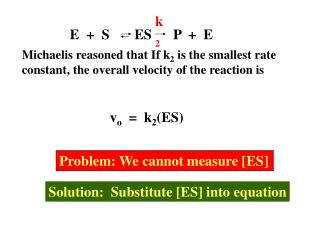# E + S ES P + E - PowerPoint PPT PresentationDownload PresentationE + S ES P + E

E + S ES P + E
Download Presentation## E + S ES P + E

- - - - - - - - - - - - - - - - - - - - - - - - - - - E N D - - - - - - - - - - - - - - - - - - - - - - - - - - -
##### Presentation Transcript

1. E + S ES P + E k2 Michaelis reasoned that If k2 is the smallest rate constant, the overall velocity of the reaction is vo = k2(ES) Problem: We cannot measure [ES] Solution: Substitute [ES] into equation

2. Evaluating and Using the Michaelis-Menten Equation

3. Vmax variables Km Dependent variable Constants pO2 vo = q = P50 + pO2 [S] Vmax [S] C + [S] Km + [S] [S] vo vo = [S] Y axis (Dependent Variable) Rectangular Hyperbola X axis (Independent variable)

4. vo = Vmax [S] Vmax [S] Vmax [S] Vmax [S] 2[S] [S] Km + [S] Km S << Km vo = First order with [S] vo = One-half Vmax S = Km vo = Zero Order with [S] S >> Km

5. Vmax = k2[ET] Vmax is first order with enzyme Slope = kcat When the velocity = Vmax k2 = kcat Vmax E4 vo E3 E2 Enzyme E1 [S]

6. vo = Vmax Vmax vo = Vmax 2 2 Vmax [S] vo = Km S S S [S] = Km S S Vmax Km = [S] at one-half Vmax

7. HIGH [S] LOW [S] Picture it this way

8. k2 + k-1 Km= k1 TWO DEFINITIONS OF KM Rate Constant Definition Affinity No units Substrate Definition Km = [S] that gives 1/2 Vmax Km = [S] that fills half the sites on the enzyme Km has units of substrate concentration

9. Calculate the Km of an enzyme. When [S] is 2 micromolar, vo = 3 micromoles per minute. At saturation Vmax = 10 micromoles per minute. What does the answer tell you? vo = 10 moles/min x 2 M 3 moles/min = Km + 2 M Vmax [S] Km + [S] Setup: 3(Km + 2) = 20 3Km + 6 = 20 3Km = 14 Km = 4.7 M When the [S] is 4.7 M, the enzyme is half-saturated with [S] When the [S] is 4.7 M, [Efree] = [ES] Km holds the same literal meaning as pKa and P50

10. Vo [S] An enzyme has a Km of 10 M. At what [S] will the reaction be at Vmax? a) 20 M b) 5 M c) cannot tell Reason: The effect of S on velocity is hyperbolic, not linear An enzyme has a Km of 10 M. When [S] equals 5 M 15 moles of S are consumed per minute. At what [S] will the reaction be at Vmax a) 20 M b) 45 M c) cannot tell What is the Vmax of the above reaction a) 20 M b) 45 moles/min c) cannot tell

11. [S] Vo = Vmax Km + [S] How close to Vmax will a reaction be when [S] = 1) Km 2) 10 Km 3) 100 Km Relative Max velocity 1) One-half Vmax 1/2 2) 90.9% Vmax 10/11 3) 99% Vmax 100/101

12. Based on the Kinetic analysis we can conclude: There are two phases of an enzyme-catalyzed reaction 1. Binding the substrate as determined by Km • Modifying the substrate and releasing the product as determined by K2 The two phases will become more apparent when we study inhibitors